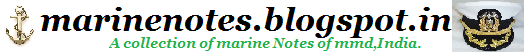Home » , , , » Types of Heat Exchangers,Parallel flow,Cross flow,Counter flow

# Types of Heat Exchangers,Parallel flow,Cross flow,Counter flow

-->

Types of Heat Exchangers
Because heat exchangers come in so many shapes, sizes, makes, and models, they are categorized according to common characteristics. One common characteristic that can be used to categorize them is the direction of flow the two fluids have relative to each other. The three categories are parallel flow, counter flow and cross flow.

Parallel flow, as illustrated in Figure 3, exists when both the tube side fluid and the shell
side fluid flow in the same direction. In this case, the two fluids enter the heat
exchanger from the same end with a large temperature difference. As the fluids transfer
heat, hotter to cooler, the temperatures of the two fluids approach each other. Note that
the hottest cold-fluid temperature is always less than the coldest hot-fluid temperature.
Counter flow, as illustrated in Figure 4, exists when the two fluids flow in opposite
directions. Each of the fluids enters the heat exchanger at opposite ends. Because the
cooler fluid exits the counter flow heat exchanger at the end where the hot fluid enters
the heat exchanger, the cooler fluid will approach the inlet temperature of the hot fluid.
Counter flow heat exchangers are the most efficient of the three types. In contrast to the
parallel flow heat exchanger, the counter flow heat exchanger can have the hottest coldfluid
temperature greater than the coldest hot-fluid temperature.
Cross flow, as illustrated in Figure 5, exists when one fluid flows perpendicular to the
second fluid; that is, one fluid flows through tubes and the second fluid passes around the
tubes at 90° angle. Cross flow heat exchangers are usually found in applications where
one of the fluids changes state (2-phase flow). An example is a steam system's
condenser, in which the steam exiting the turbine enters the condenser shell side, and the
cool water flowing in the tubes absorbs the heat from the steam, condensing it into water.
Large volumes of vapor may be condensed using this type of heat exchanger flow.
Comparison of the Types of Heat Exchangers
Each of the three types of heat exchangers has advantages and disadvantages. But of the three, the counter flow heat exchanger design is the most efficient when comparing heat transfer rate per unit surface area. The efficiency of a counter flow heat exchanger is due to the fact that the average   T (difference in temperature) between the two fluids over the length of the heat exchanger is maximized, as shown in Figure 4. Therefore the log mean temperature for a
counter flow heat exchanger is larger than the log mean temperature for a similar parallel or
cross flow heat exchanger. (See the Thermodynamics, Heat Transfer, and Fluid Flow
Fundamentals Handbook for a review of log mean temperature). This can be seen by comparing the graphs in Figure 3, Figure 4, and Figure 5. The following exercise demonstrates how the higher log mean temperature of the counter flow heat exchanger results in a larger heat transfer rate. The log mean temperature for a heat exchanger is calculated using the following equation.
In actuality, most large heat exchangers are not purely parallel flow, counter flow, or cross flow;
they are usually a combination of the two or all three types of heat exchangers. This is due to
the fact that actual heat exchangers are more complex than the simple components shown in theidealized figures used above to depict each type of heat exchanger. The reason for the
combination of the various types is to maximize the efficiency of the heat exchanger within the
restrictions placed on the design. That is, size, cost, weight, required efficiency, type of fluids,
operating pressures, and temperatures, all help determine the complexity of a specific heat
exchanger.One method that combines the characteristics of two or more heat exchangers and improves the performance of a heat exchanger is to have the two fluids pass each other several times within a single heat exchanger. When a heat exchanger's fluids pass each other more than once, a heat exchanger is called a multi-pass heat exchanger. If the fluids pass each other only once, the heat exchanger is called a single-pass heat exchanger. See Figure 6 for an example of both types. Commonly, the multi-pass heat exchanger reverses the flow in the tubes by use of one or more sets of "U" bends in the tubes. The "U" bends allow the fluid to flow back and forth across the length of the heat exchanger. A second method to achieve multiple passes is to insert baffles on the shell side of the heat exchanger. These direct the shell side fluid back and forth across the tubes to achieve the multi-pass effect.
Heat exchangers are also classified by their function in a particular system. One common
classification is regenerative or nonregenerative. A regenerative heat exchanger is one in which the same fluid is both the cooling fluid and the cooled fluid, as illustrated in Figure 7. That is, the hot fluid leaving a system gives up its heat to "regenerate" or heat up the fluid returning to
the system. Regenerative heat exchangers are usually found in high temperature systems where a portion of the system's fluid is removed from the main process, and then returned. Because the fluid removed from the main process contains energy (heat), the heat from the fluid leaving the main system is used to reheat (regenerate) the returning fluid instead of being rejected to an external cooling medium to improve efficiency. It is important to remember that the term regenerative/nonregenerative only refers to "how" a heat exchanger functions in a system, and does not indicate any single type (tube and shell, plate, parallel flow, counter flow, etc.).
In a nonregenerative heat exchanger, as illustrated in Figure 7, the hot fluid is cooled by fluid
from a separate system and the energy (heat) removed is not returned to the system.-->